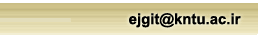[Home ] [Archive]   [ فارسی ]Volume 4, Issue 3 (12-2016)jgit 2016, 4(3): 107-119 Back to browse issues page
On the regional gravity field modeling via Radial Basis Functions and modefied Levenberg-Marcoardet Algorithm
University of Tehran
Abstract:   (3243 Views)

The gravity field modeling can be performed in global or local scales utilizing satellite, airborne, terrestrial gravity data or a combination of these observations. One of the common methods in gravity field approximation is to use Spherical Harmonic expansion. Due to the global characteristics of the Spherical Harmonic base functions, a small local signal variation can change all the coefficients in the expansion, and therefore, they are not a suitable choice for regional applications. In order to overcome this problem, there are several groups of base functions, including Radial Basis Functions (RBFs). In gravity field modeling using RBFs, the disturbing potential is represented by a linear combination of an infinite set of RBFs. Hence, any linear functional of the disturbing potential, such as gravity anomaly or gravity disturbance, can be also expressed based on the RBF expansion. Thus, measurable quantities of the Earth's gravity field can be utilized in order to determine the RBF parameters in gravimetric modeling. In this study, system of observation equations is set based on the Radial Multi-Poles of order 2 and free-air gravity anomalies and unknown parameters, including RBF centers, RBF bandwidths (or depths) and scaling coefficients, are determined using a least-squares method. In fact, the Levenberg-Marquardt algorithm is applied as a non-linear regularization method to simultaneously optimize all the RBF parameters. In order to enhance the numerical efficiency of this algorithm, a novel scheme is proposed to initialize and update the regularization parameter. Finally, numerical results obtained from the modified Levenberg-Marquardt algorithm are compared with the ones obtained from the simple form of this algorithm. Applying the proposed modifications to this algorithm, the unknown parameters are determined in a fast procedure and with a significant reduction in the number of iterations. Moreover, these modifications can increase the probability of convergence of the solution to the global minimum.

Keywords: Radial Base Function, Radial multipole kernel, Regional gravity field modeling, nonlinear inverse problem, Levenberg-Marquardt algorithm.
Type of Study: Research | Subject: Geodesy
Received: 2016/04/12 | Accepted: 2016/07/20 | Published: 2017/02/28
Send email to the article author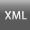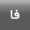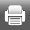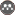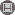Rights and permissionsThis work is licensed under a Creative Commons Attribution-NonCommercial 4.0 International License.